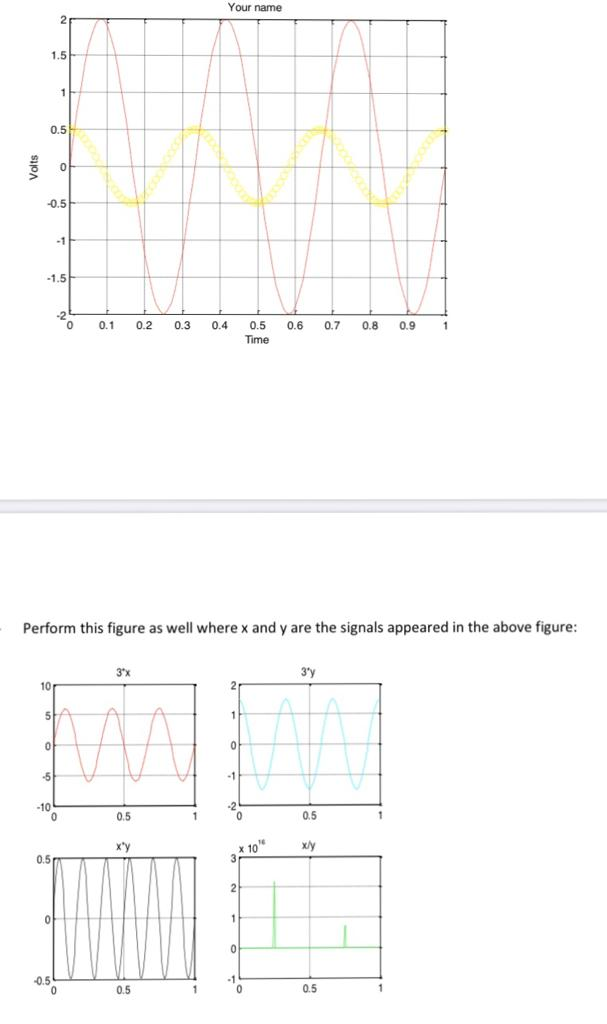# how to write a code to get these figures ??

1 view (last 30 days)
mike john on 21 Apr 2020
Commented: Rena Berman on 1 Jun 2020Show 1 older comment
John D'Errico on 24 May 2020
Stop deleting your question. If you don't want it on Answers, then you should never post in the first place. Deleting the question is an insult to the person who bothered to answer your question.
Stephen Cobeldick on 24 May 2020
Original question by mike John recovered from Google Cache:
"how to write a code to get these figures ??"Rena Berman on 1 Jun 2020

KSSV on 21 Apr 2020
Edited: KSSV on 21 Apr 2020
th = linspace(0,2*pi) ;
x1 = sin(th) ;
x2 = cos(th) ;
figure(1)
plot(th,x1)
figure(2)
plot(th,x1,'r') ;
hold on
plot(th,x2,'b') ;
figure(3)
subplot(121)
plot(th,x1)
subplot(122)
plot(th,x2)

BALAJI KARTHEEK on 21 Apr 2020
this code will help you out, but i am not sure about the graph of fourth figure but x/y yields to tan graph that is what i am getting since x is sin function and y is cos function
clc
clear all
close all
a1=2;
a2=0.5;
t=0:0.01:1;
T=0.33;
y1=a1*sin(2*pi*t/T);
y2=a2*cos(2*pi*t/T);
figure
plot(t,y1)
hold on
plot(t,y2,'o')
grid on
ylabel('Time')
xlabel('Volts')
figure
subplot(2,2,1)
plot(t,3*y1)
title('3*x')
subplot(2,2,2)
plot(t,3*y2)
title('3*y')
subplot(2,2,3)
plot(t,y2.*y1)
title('x*y')
subplot(2,2,4)
plot(t,y1./y2)
title('x/y')